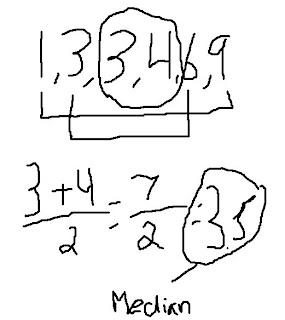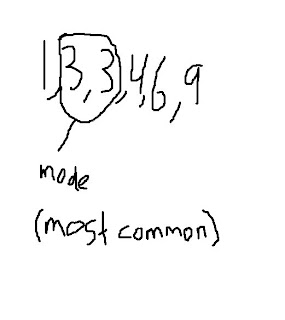### Adrian's Mesures Of Central Tendency

Wednesday, October 1, 2008
- The sum of all numbers divided by the number of data.Median
- The middle of the set of numbers in ascending numerical order.Mode - The most common number in a set of numbers in ascending numerical order.Range - The biggest number subtracted by the smallest number in a set of data in ascending
numerical order.Here's a video for measures of central tendency